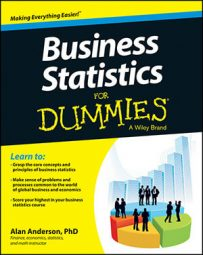When drawing conclusions about a population from randomly chosen samples (a process called statistical inference), you can use two methods: confidence intervals and hypothesis testing.

## Confidence intervals

A confidence interval is a range of values that's expected to contain the value of a population parameter with a specified level of confidence (such as 90 percent, 95 percent, 99 percent, and so on). For example, you can construct a confidence interval for the population mean by following these steps:

1. Estimate the value of the population mean by calculating the mean of a randomly chosen sample (known as the sample mean).

2. Calculate the lower limit of the confidence interval by subtracting a margin of error from the sample mean.

3. Calculate the upper limit of the confidence interval by adding the same margin of error to the sample mean.

The margin of error depends on the size of the sample used to construct the confidence interval, whether the population standard deviation is known, and the level of confidence chosen.

The resulting interval is known as a confidence interval. A confidence interval is constructed with a specified level of probability. For example, suppose you draw a sample of stocks from a portfolio, and you construct a 95 percent confidence interval for the mean return of the stocks in the entire portfolio:

(lower limit, upper limit) = (0.02, 0.08)

The returns on the entire portfolio are the population of interest. The mean return in each sample drawn is an estimate of the population mean. The sample mean will be slightly different each time a new sample is drawn, as will the confidence interval. If this process is repeated 100 times, 95 of the resulting confidence intervals will contain the true population mean.

## Hypothesis testing

Hypothesis testing is a procedure for using sample data to draw conclusions about the characteristics of the underlying population.

The procedure begins with a statement, known as the null hypothesis. The null hypothesis is assumed to be true unless strong evidence against it is found. An alternative hypothesis — the result accepted if the null hypothesis is rejected — is also stated.

You construct a test statistic, and you compare it with a critical value (or values) to determine whether the null hypothesis should be rejected. The specific test statistic and critical value(s) depend on which population parameter is being tested, the size of the sample being used, and other factors.

If the test statistic is too extreme (for example, it's too large compared with the critical value[s]) the null hypothesis is rejected in favor of the alternative hypothesis; otherwise, the null hypothesis is not rejected.

If the null hypothesis isn't rejected, this doesn't necessarily mean that it's true; it simply means that there is not enough evidence to justify rejecting it.

Hypothesis testing is a general procedure and can be used to draw conclusions about many features of a population, such as its mean, variance, standard deviation, and so on.